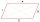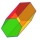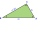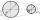# Triangle + angle - math problems

#### Number of problems found: 394

• Find theFind the content of a regular 12 sided polygon, if its side a = 12 cm.
• Four sides of trapezoidIn the trapezoid ABCD is |AB| = 73.6 mm; |BC| = 57 mm; |CD| = 60 mm; |AD| = 58.6 mm. Calculate the size of its interior angles.
• Right triangleCalculate the length of the remaining two sides and the angles in the rectangular triangle ABC if a = 10 cm, angle alpha = 18°40'.
• Trapezium internal anglesA trapezium where AB is parallel to CD, has angle A : angle D = 4 :5, angle B = 3x-15 and angle C = 4x+20. Find angle A, B, C and D.
• Two chordsFrom the point on the circle with a diameter of 8 cm, two identical chords are led, which form an angle of 60°. Calculate the length of these chords.
• Five circlesOn the line segment CD = 6 there are 5 circles with radius one at regular intervals. Find the lengths of the lines AD, AF, AG, BD, and CE
• As shownAs shown, in △ ABC, ∠C = 90°, AD bisects ∠BAC, DE⊥AB to E, BE = 2, BC = 6, then the perimeter of △ BDE
• Tetrahedral pyramid 8Let’s all side edges of the tetrahedral pyramid ABCDV be equally long and its base let’s be a rectangle. Determine its volume if you know the deviations A=40° B=70° of the planes of adjacent sidewalls and the plane of the base and the height h=16 of the p
• IsoscelesIsosceles trapezium ABCD ABC = 12 angle ABC = 40 ° b=6. Calculate the circumference and area.
• HexagonCalculate the surface of a regular hexagonal prism whose base edge a = 12cm and side edge b = 3 dm.
• Acute triangleIn the acute triangle KLM, V is the intersection of its heights and X is the heel of height to the side KL. The axis of the angle XVL is parallel to the side LM and the angle MKL is 70°. What size are the KLM and KML angles?
• Angle of two linesThere is a regular quadrangular pyramid ABCDV; | AB | = 4 cm; height v = 6 cm. Determine the angles of lines AD and BV.
• V-beltCalculate a length of the V-belt when the diameter of the pulleys is: D1 = 600 mm D2 = 120 mm d = 480 mm
• ABCDAC= 40cm , angle DAB=38 , angle DCB=58 , angle DBC=90 , DB is perpendicular on AC , find BD and AD

We apologize, but in this category are not a lot of examples.
Do you have an interesting mathematical word problem that you can't solve it? Submit a math problem, and we can try to solve it.

We will send a solution to your e-mail address. Solved examples are also published here. Please enter the e-mail correctly and check whether you don't have a full mailbox.

Please do not submit problems from current active competitions such as Mathematical Olympiad, correspondence seminars etc...